# Exploration of Physics Students Mathematical Difficulties David E

• Slides: 19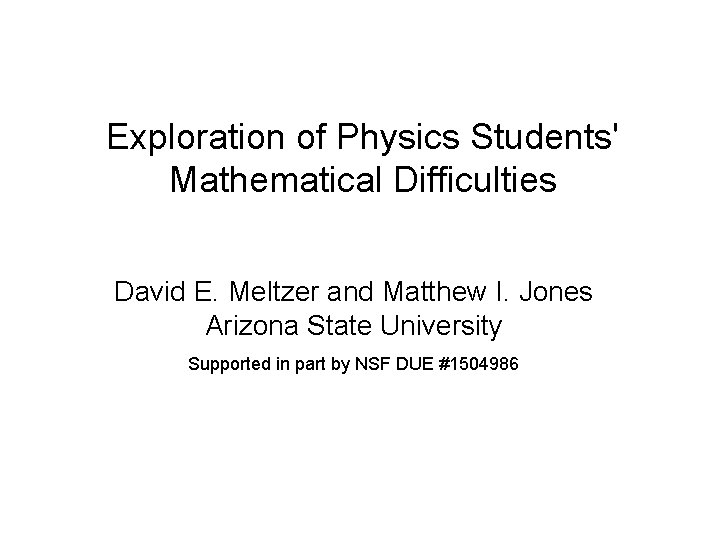Exploration of Physics Students' Mathematical Difficulties David E. Meltzer and Matthew I. Jones Arizona State University Supported in part by NSF DUE #1504986The Problem • Difficulties with very basic math skills impact performance of introductory physics students. • The difficulties are often not resolved by students’ previous mathematical training. • Students can’t effectively grapple with physics ideas when they feel overburdened in dealing with calculational issues.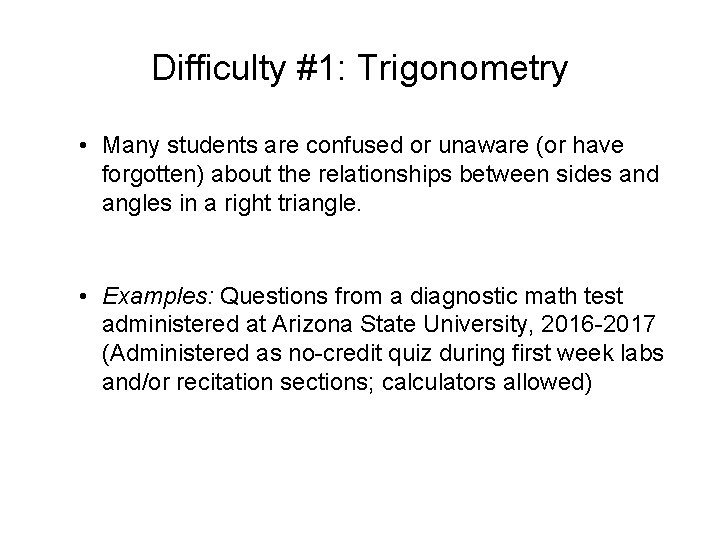Difficulty #1: Trigonometry • Many students are confused or unaware (or have forgotten) about the relationships between sides and angles in a right triangle. • Examples: Questions from a diagnostic math test administered at Arizona State University, 2016 -2017 (Administered as no-credit quiz during first week labs and/or recitation sections; calculators allowed)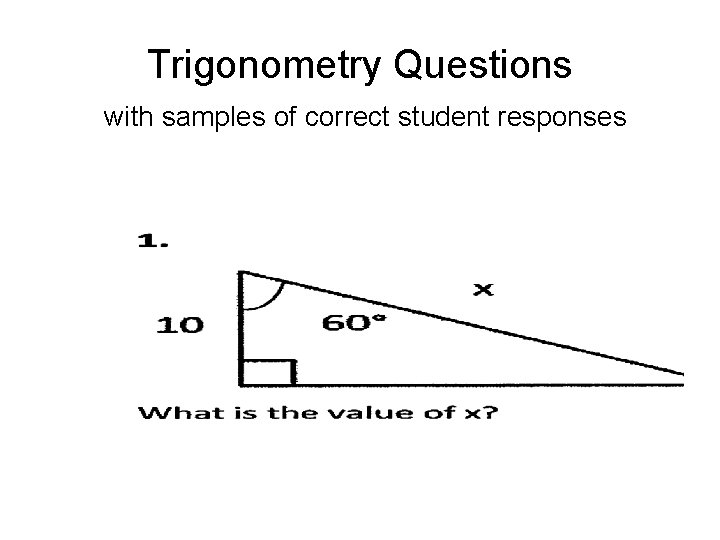Trigonometry Questions with samples of correct student responses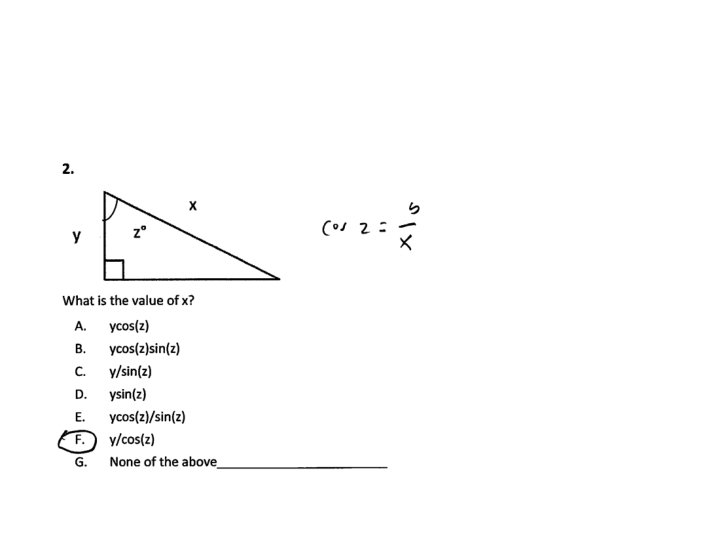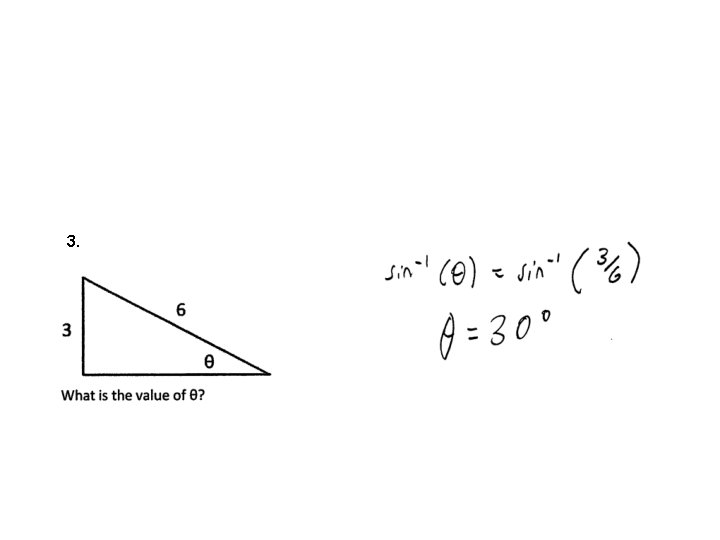3.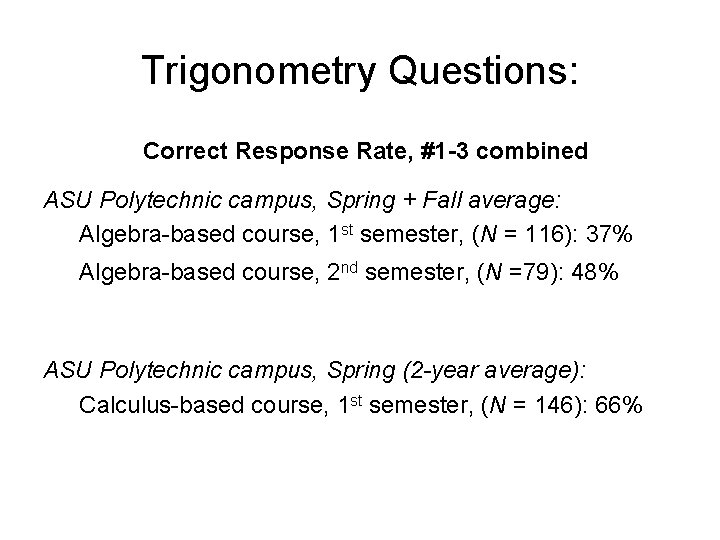Trigonometry Questions: Correct Response Rate, #1 -3 combined ASU Polytechnic campus, Spring + Fall average: Algebra-based course, 1 st semester, (N = 116): 37% Algebra-based course, 2 nd semester, (N =79): 48% ASU Polytechnic campus, Spring (2 -year average): Calculus-based course, 1 st semester, (N = 146): 66%Results on Trigonometry Questions Errors oberved: use of incorrect trigonometric function (e. g. , cosine instead of sine), calculator set on radians instead of degrees, algebra errors; unaware (or forgot) about inverse trigonometric functions, e. g. , arctan, arcsin, arccos [tan-1, sin-1, cos-1] – How to address these problems: It seems that students require substantial additional practice and repetition with basic trigonometric proceduresTrigonometry Questions: Polytechnic/Tempe Campus Difference Error Rate (% incorrect responses) Algebra-based course, second semester; #1 -3 combined: ASU Polytechnic campus, Spring (N = 52): 59% ASU Tempe campus, Spring (N = 61): 35%Trigonometry Questions: Spring/Fall Semester Difference Error Rate (% incorrect responses) Algebra-based course, first semester; #1 -3 combined: ASU Polytechnic campus, Spring (N = 72): 67% ASU Polytechnic campus, Fall (N = 44): 58% Algebra-based course, second semester; #1 -3 combined: ASU Polytechnic campus, Spring (N = 52): 59% ASU Polytechnic campus, Fall (N = 27): 44% Calculus-based course, first semester; #1 only: ASU Polytechnic campus, Spring (N = 104): 40% ASU Polytechnic campus, Fall (N = 98): 56%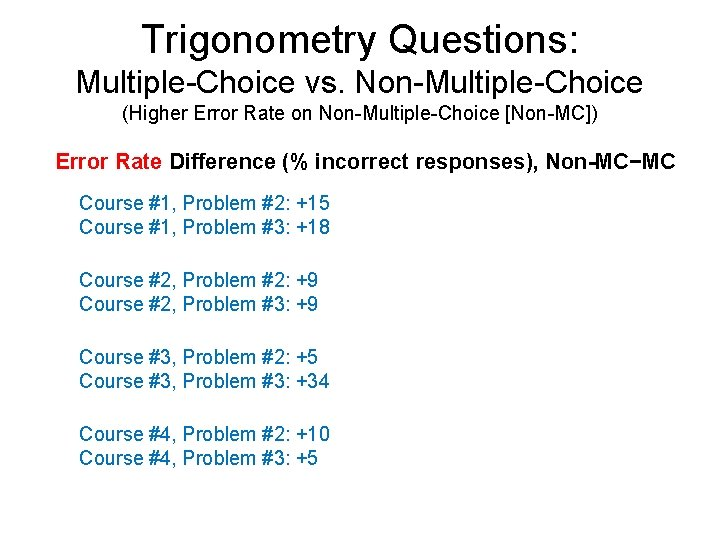Trigonometry Questions: Multiple-Choice vs. Non-Multiple-Choice (Higher Error Rate on Non-Multiple-Choice [Non-MC]) Error Rate Difference (% incorrect responses), Non-MC−MC Course #1, Problem #2: +15 Course #1, Problem #3: +18 Course #2, Problem #2: +9 Course #2, Problem #3: +9 Course #3, Problem #2: +5 Course #3, Problem #3: +34 Course #4, Problem #2: +10 Course #4, Problem #3: +5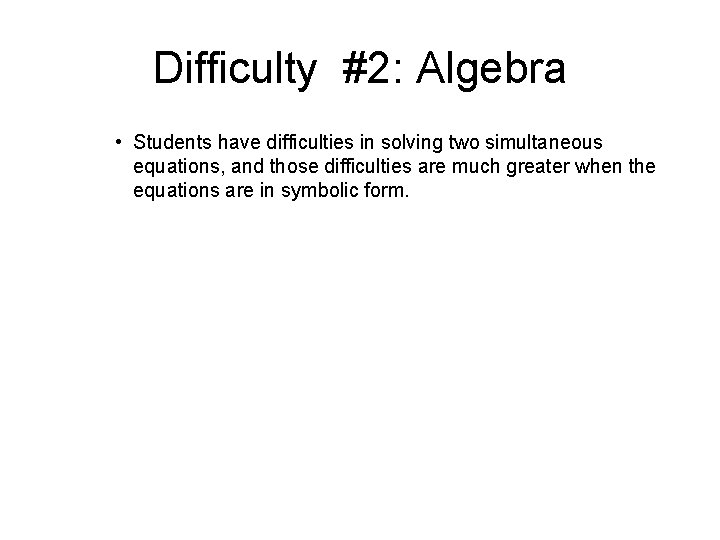Difficulty #2: Algebra • Students have difficulties in solving two simultaneous equations, and those difficulties are much greater when the equations are in symbolic form.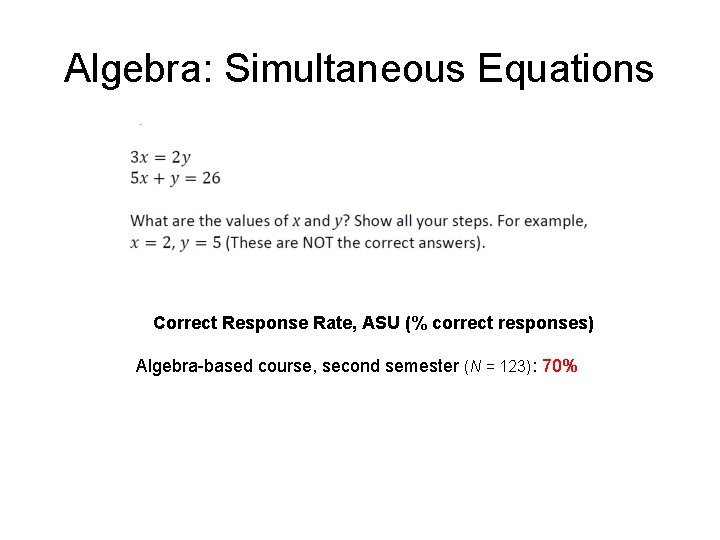Algebra: Simultaneous Equations Correct Response Rate, ASU (% correct responses) Algebra-based course, second semester (N = 123): 70%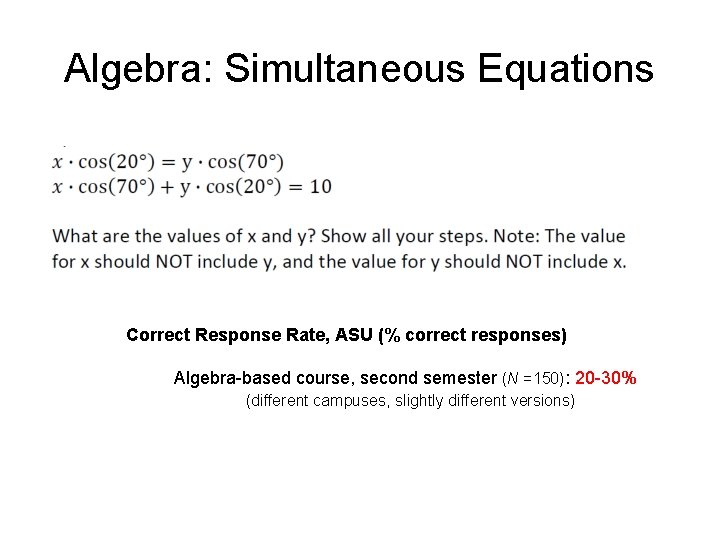Algebra: Simultaneous Equations Correct Response Rate, ASU (% correct responses) Algebra-based course, second semester (N =150): 20 -30% (different campuses, slightly different versions)Algebra: Simultaneous Equations Correct Response Rate, ASU (% correct responses) Algebra-based course, second semester (N =150): 10 -20% (different campuses, slightly different versions) Only 10 -20% correct responses!Algebra: Simultaneous Equations Sample of Correct Student ResponseSources of Difficulties • Carelessness – Students very frequently self-correct errors during interviews – Evidence of carelessness on written diagnostic • Skill practice deficit: Insufficient repetitive practice with learned skills – E. g. , applying definitions of sine and cosine; factoring out variables in algebraic expressions • Conceptual confusion – E. g. , not realizing that sides and angles of right triangle are related by trigonometric functions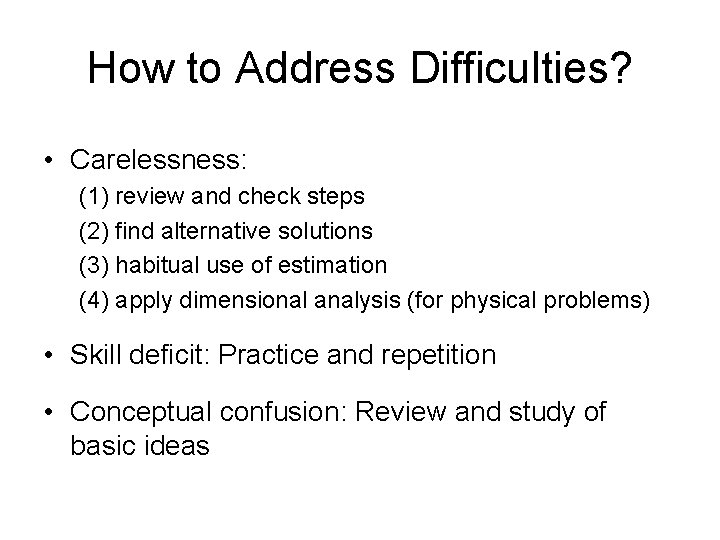How to Address Difficulties? • Carelessness: (1) review and check steps (2) find alternative solutions (3) habitual use of estimation (4) apply dimensional analysis (for physical problems) • Skill deficit: Practice and repetition • Conceptual confusion: Review and study of basic ideas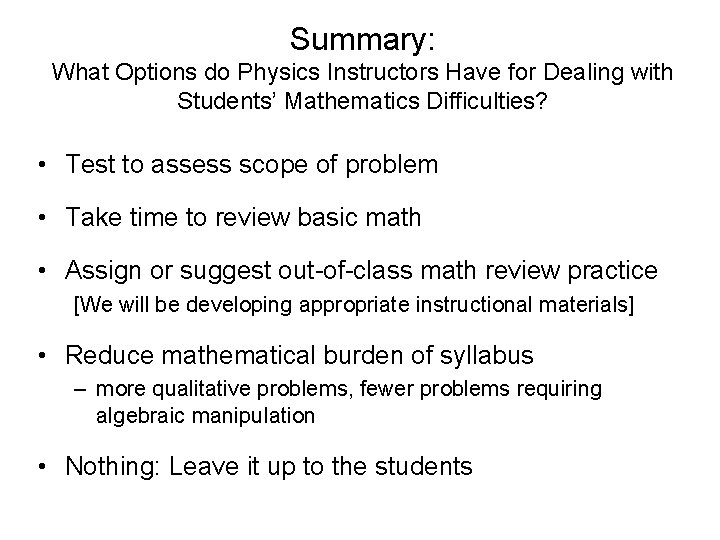Summary: What Options do Physics Instructors Have for Dealing with Students’ Mathematics Difficulties? • Test to assess scope of problem • Take time to review basic math • Assign or suggest out-of-class math review practice [We will be developing appropriate instructional materials] • Reduce mathematical burden of syllabus – more qualitative problems, fewer problems requiring algebraic manipulation • Nothing: Leave it up to the students